# Brown-Gitler spectra

(diff) ← Older revision | Latest revision (diff) | Newer revision → (diff)

Spectra introduced by E.H. Brown Jr. and S. Gitler [a1] to study higher-order obstructions to immersions of manifolds (cf. also Immersion; Spectrum of spaces). They immediately found wide applicability in a variety of areas of homotopy theory, most notably in the stable homotopy groups of spheres ([a9] and [a4]), in studying homotopy classes of mappings out of various classifying spaces ([a3], [a10] and [a8]), and, as might be expected, in studying the immersion conjecture for manifolds ([a2] and [a5]).

The modulo-homology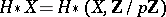comes equipped with a natural right action of the Steenrod algebrawhich is unstable: at the prime, for example, this means that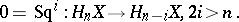Write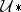for the category of all unstable right modules over. This category has enough projective objects; indeed, there is an object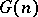,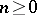, ofand a natural isomorphism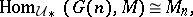where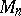is the vector spaces of elements of degreein. The modulecan be explicitly calculated. For example, if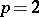and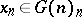is the universal class, then the evaluation mappingsendingto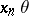defines an isomorphism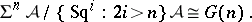These are the dual Brown–Gitler modules.

This pleasant bit of algebra can be only partly reproduced in algebraic topology. For example, for generalthere is no space whose (reduced) homology is; specifically, if, the module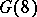cannot support the structure of an unstable co-algebra over the Steenrod algebra. However, after stabilizing, this objection does not apply and the following result from [a1], [a4], [a6] holds: There is a unique-complete spectrumso that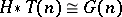and for all pointed CW-complexes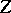, the mapping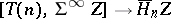sendingto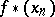is surjective. Here,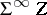is the suspension spectrum of, the symboldenotes stable homotopy classes of mappings, and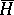is reduced homology. The spectraare the dual Brown–Gitler spectra. The Brown–Gitler spectra themselves can be obtained by the formula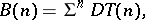wheredenotes the Spanier–Whitehead duality functor. The suspension factor is a normalization introduced to put the bottom cohomology class ofin degree. An easy calculation shows that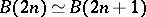for all prime numbers and all.

For a general spectrumandmodulo, the group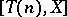is naturally isomorphic to the groupof homogeneous elements of degreein the Cartier–Dieudonné module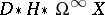of the Abelian Hopf algebra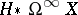. In fact, one way to construct the Brown–Gitler spectra is to note that the functor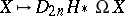is the degree-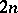group of an extraordinary homology theory; thenis the-completion of the representing spectrum. See [a6]. This can be greatly, but not completely, destabilized. See [a7].

How to Cite This Entry:
Brown-Gitler spectra. Encyclopedia of Mathematics. URL: http://encyclopediaofmath.org/index.php?title=Brown-Gitler_spectra&oldid=17469
This article was adapted from an original article by Paul Goerss (originator), which appeared in Encyclopedia of Mathematics - ISBN 1402006098. See original article